# Multiplication - 6th grade (11y) - math problems

#### Number of problems found: 352

• Countertop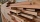Sam it is constructing he measures a rectangle area are on the countertop of 18 1/2 x 35 1/3” he will need to cut his area out in order to place his sink and the opening. What is the product of the two measurements round to the nearest whole?
• Francesca 2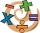Francesca applied the steps below to find the product of (-1.2)(-9.4). Step 1: (-1.2)(-9.4) = (-9.4)(-1.2) Step 2:                   = (-9.4)(-1) + (-9.4)(-0.2) Step 3:                   = (9.4) + (1.88) Step 4:                   = 11.28 Which step shows
• Mother 7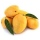Mother bought 18 fruits. 1/3 were pineapple and the rest  were mangoes . how many were mangoesAdd six to twelve, then divide the sum by four. Divide the quotient by negative nine and then multiply by twenty-four. What is the result?
• If Ben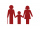If Ben is ⅕ the age of his father, ¼ the age of his mother, and twice the age of his sister, how old are his parents and sister if he is 8 years old?
• Product and sumWhat is the product of two fourths  and the sum of three halves and four?About 6/9 of the sixth- grade pupils will be going to the parents' seminar. If 1/6 of the participants are girls, what part of the portion of sixth graders are boys?
• The libraryThere are 65 boxes of books. Each box contains 100 books. If each book costs 19.75 how much is the total cost of all the books?
• In dividingIn dividing fractions, get the reciprocal of the divisor and change division symbol to multiplication symbol. 2/3 : 5/6
• The space museum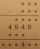The science club is going to the space museum. The club consists of 27 students. If admission costs \$6.50 for children ages 4–11 and \$8.50 for children ages 12–17, how much will it cost for the entire science club's admission to the museum? Science Club S
• Nathan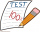Nathan is playing a trivia game. Each correct answer is worth 2 ½ points, and each incorrect answer is worth -¼ points. If he gets 35 questions correct and 32 questions incorrect, how many points does he have? A. 87.5 B. 79.5 C. 75 D. 8
• A giraffe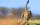A giraffe can run up to 46.93 feet per second. How far could a giraffe run in 1.8 seconds?
• 30 eggs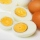There are 30 eggs in a tray. If 1/2 of the tray used 1/5 of it cooked,1/3 kept the refrigerator, how many eggs were left?
• Translate 2Translate the given phrases to mathematical phrases. Thrice the sum of three fifths and two thirds less one half is what number?
• Yellow tangs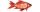In an aquarium 2/5 of the fish are surgeonfish.  Of these 3/4 are yellow tangs.  What fraction of all fish in the aquarium are yellow tangs?
• A land 2A land owner owns two parcels of land measuring 4 2/5 hectares and 6 1/5 hectares. He sells 3/4 of the total land. How many hectares of the land are left?
• AlexandraAlexandra made a rectangular quilt the measured 3 1/4. 2 3/4 feet in width. What is the area of the quilt in square feet? Write an equation to solve.
• Evaluate 17Evaluate 2x+6y when x=- 4/5 and y=1/3. Write your answer as a fraction or mixed number in simplest form.
• A burger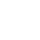The water starts with 41 1/4 cups of water how much water is left after ten 3 5/8 Scoops of water are removed?
• English and math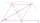1/5 of a class study English, and 3/5 study mathematics. If the class consist of 80 students, how many students study English and mathematics in the class?

Do you have an exciting math question or word problem that you can't solve? Ask a question or post a math problem, and we can try to solve it.

We will send a solution to your e-mail address. Solved examples are also published here. Please enter the e-mail correctly and check whether you don't have a full mailbox.